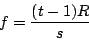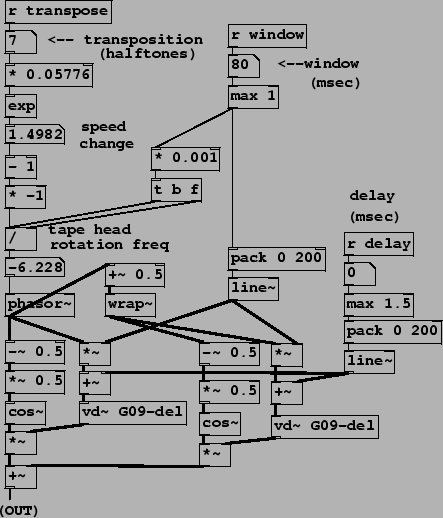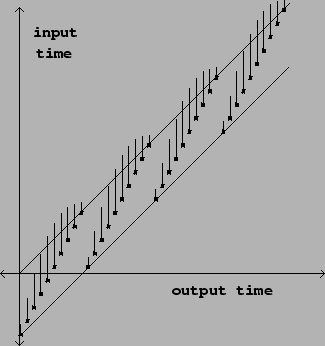•ricky

I thought the cosine shape was really just convenience given that [cos~] is available and that allows for a sample-accurate solution that doesn't involve reading from a table.

posted in technical issues read more
•ricky

@whale-av said:

@katjav gave it a lot more thought here........ https://www.katjaas.nl/pitchshift/pitchshift.html
I could be wrong but I don't see why the samplerate would be part of the calculation (it isn't)...... as all variables are relative.

I was confused because R represents sample rate earlier in the text.

"If the frequency of the sawtooth wave is \$f\$ (in cycles per second), then its value sweeps from 0 to 1 every \$R/f\$ samples (where \$R\$ is the sample rate)."

posted in technical issues read more
•ricky

@whale-av said:

@ricky It's [- 1] because the exponent of 0 (no shift) is 1 but the tape head has to be stationary (no rotation = 0) for playback at normal speed......

Ah, thanks. That makes sense. Thanks, David.

And [* -1] because (although it seems wrong until you really think about it) the tape head has to be turning backwards relative to the tape (negative values for [phasor~]...) for the pitch to increase (positive transposition values).
There is no point arguing with the patch because it works as expected.......Who is arguing?This was my understanding.

[cos~] windows the output so that amplitude sums are "sort of" ok (with the in and out of phase bits) and the vertical edge of the [phasor~] saw is declicked.

Yes, a nicely scaled fade in and out.

posted in technical issues read more
•ricky

Cocktail hour is the best hour. Good man and good catch! I totally forgot about the 0.001 multiplier and of course it factors it out. D'oh. As for the [* -1], I thought this made sense in the context of the Pd patch given the positive ramp of the phasor and without it the pitch transposition step is inverted.

What you're saying is that the algebra below is incorrect? Maybe it has something to do with the way the transposition factor is calculated?It's too early for a cocktail here. Still on my first coffee.

posted in technical issues read more
•ricky

@jameslo - I remembered this live granular synthesis example from the Pd tutorial website which makes sense to me.

The transposition factor is handled as `pow(2, t/12) - 1 * 44100/s)` where t is the transposition step and s is the windows size. Maybe we can reconcile the differences and figure out how Miller factor the sample rate.

posted in technical issues read more
•ricky

So, you're saying the `-1` is in place to ensure we get decreasing delay amounts for when we want to pitch up and increasing delay amounts when we want to pitch down because of the nature of phasor~? That makes sense but it doesn't explain the `R` in the math.

posted in technical issues read more
•ricky

Cool. Thanks, @jameslo.

So, getting to the Pitch Shifter example itself, it's easy enough to follow the transposition factor from the math to patch but I'm wondering why the -1 preceding the tape head rotation frequency isn't the current sample rate, as per the math?`f = (t - 1) * R / s`

I see where one is subtracted from t and where the window size is divided into that but why does R = -1? Is this some other interpretation of 'sample rate?'

posted in technical issues read more
•ricky

Ah! So `d[n]` is basically the distance between the original input signal (no delay) and the variable delay.

So the output sample here would be at the origin diagonal at the bottom of the blue vertical line as you've drawn it?

Would I be correct in saying that the variable delay line is pitching down at that intersection in the illustration you've modified?I'd still love to know if when sample points in the diagram above are representative of a decreasing delay line time over each period and if there is continuity between each ascending sample (which would pitch shift our input signal up, as I understand it?) this would lead to a continuous pitch shift? My intuition says yes but it's fun to talk about these things, I think.

I guess that's what Miller means by, "If `d[n]` does not change with n, the transposition factor is 1 and the sound emerges from the delay line at the same speed as it went in. But if the delay time is increasing as a function of n, the resulting sound is transposed downward, and if d[n] decreases, upward."

posted in technical issues read more
•ricky

@jameslo - actually one small follow-up from your comment about the index via `n-d[n]`. I guess I am not sure how that would be read graphically. I get that we arrive at a new index value through subtraction but where does `n` intersect on this graph? Where does `d[n]` intersect? Hopefully that question makes sense. It might help to plug in some values.

posted in technical issues read more
•ricky

@jameslo, Thanks! It is super helpful to talk through it with someone else. My understanding is aligned with yours. I am relievedThe parallel line description is precisely where I had arrived when reading through this material, so thanks for confirming. I have some follow-ups so hopefully you're game to keep the conversation going. I am looking at the pitch shifting page.

This graphic is a bit confusing to me, too. Or at least I'm not sure if I am reading it correctly.How does the vertical line above each point actually relate to enveloping? Additionally, I am unsure how their trajectory actually results in a continuous pitch shift. In this illustration, I think the sample points are representative of a decreasing delay line time over each period and if there is continuity between each ascending sample (which would pitch shift our input signal up, as I understand it?) this would lead to a continuous pitch shift?

I'm trying to understand more clearly how these graphics actually relate to the idea of Momentary Transposition as it's described in the book.

posted in technical issues read more

Internal error.

Oops! Looks like something went wrong!